# What Is A Rational Function? (16 Common Questions Answered)

We often see rational functions in calculus, since some of them display interesting behavior with multiple asymptotes.  However, there is a lot more to know about rational functions.

So, what is a rational function?  A rational function is a fraction where the numerator and denominator are both polynomials. So, a rational function has the form R(x) = P(x) / Q(x), where P(x) and Q(x) are polynomials and Q(x) is not zero. If Q(x) is a constant polynomial (a horizontal line), then R(x) is a polynomial function.

Of course, rational functions can have more than one vertical asymptote, or none at all, depending on the denominator function Q(x).

In this article, we’ll talk about rational functions and what they are.  We’ll also answer some common questions about rational functions and give some examples to show the ideas clearly.

Let’s get started.

## What Is A Rational Function?

A rational function is a fraction where the numerator and denominator are both polynomials.  A rational function R(x) has the following form:

• R(x) = P(x) / Q(x)

where P(x) and Q(x) are polynomials and Q(x) is not zero.

The numerator and denominator polynomials in a rational function can have coefficients in any field, including:

• complex numbers
• real numbers
• rational numbers
• integers

In some special cases, a rational function can be a polynomial.

### Is A Rational Function A Polynomial?

A rational function R(x) is a polynomial when the denominator Q(x) is a constant polynomial (its graph would be a horizontal line).

If Q(x) = 1, then R(x) would equal P(x).  In that case, R(x) is a polynomial function with coefficients in the same field as P(x).

So, every polynomial is a rational function, but not every rational function is a polynomial.

#### Example: A Rational Function That Is A Polynomial

Consider the rational function with P(x) = x + 1 and Q(x) = 2.  Then the rational function is given by:

• R(x) = P(x) / Q(x)
• R(x) = (x + 1) / 2
• R(x) = (x / 2) + (1 / 2)

In this case, P(x) and Q(x) are both polynomials whose coefficients are integers.  R(x) is a polynomial whose coefficients are rational numbers.

### Can A Rational Function Have A Square Root?

A rational function cannot have a square root in the numerator or denominator.  The reason is the definition of a rational function: it is a quotient of polynomial functions.

For a rational function R(x) = P(x) / Q(x), both P(x) and Q(x) are polynomials.  This means that they contain terms with variables raised to positive integer powers.

So, there are no fractions, decimals, or negative numbers in the exponents of the terms in a polynomial.  Since a square root is the same as an exponent of ½ (that is, √x = x1/2), we know that a polynomial cannot have a square root.

By similar reasoning, a rational function cannot have a cube root, fourth root, or any other root in the numerator or denominator, since:

• 3√x = x1/3
• 4√x = x1/4
• 5√x = x1/5
• etc…

### Can Rational Functions Have Fractional Exponents?

Rational functions cannot have fractional exponents.  As mentioned earlier, the reason is the definition of a rational function: it is a quotient of polynomial functions.

For a rational function R(x) = P(x) / Q(x), both P(x) and Q(x) are polynomials.  This means that they contain terms with variables raised to positive integer powers.

So, there are no fractions in the exponents of the terms in a polynomial.

### How To Graph A Rational Function

Let’s take a look at how to graph a rational function by using its asymptotes.

#### Example: Graphing A Rational Function

Consider the rational function:

• R(x) = (x – 2) / (x + 3)

This is a rational function, since the numerator is the polynomial P(x) = x – 2 and the denominator is the polynomial Q(x) = x + 3.

Before graphing, we find asymptotes as follows:

• Vertical asymptotes: set the denominator equal to zero and solve for x.  This gives us Q(x) = 0, or x + 3 = 0, which means x = -3 is a vertical asymptote of this rational function.
• Horizontal and skew asymptotes: take the limit of R(x) as x approaches positive infinity and as x approaches negative infinity to find the horizontal asymptotes.  Here, we get y = 1 as a horizontal asymptote.

Now we can start to sketch our graph.  First, we add the vertical asymptote x = -3 (the dotted blue vertical line):

Next, we add the horizontal asymptote y = 1 (the dotted red horizontal line):

Then, we figure out what the graph looks like to the left of the vertical asymptote (for x < -3).  We can use a test value of x = -4 to find:

• R(x) = (x – 2) / (x + 3)
• R(-4) = (-4 – 2) / (-4 + 3)
• R(-4) = (-6) / (-1)
• R(-4) = 6

So the point (-4, 6) is on the graph of R(x).  This means that the function lies above the horizontal asymptote y = 1 to the left of the vertical asymptote x = -3: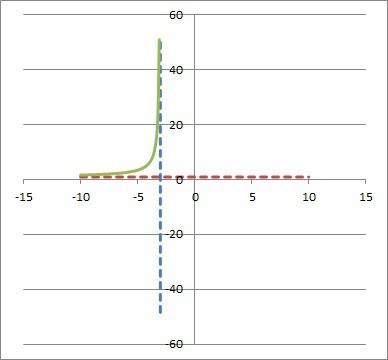Then, we graph the left half of the rational function R(x) = (x – 2) / (x + 3), for x < -3 (green curve).

Finally, we figure out what the graph looks like to the right of the vertical asymptote (for x > -3).  We can use a test value of x = 0 to find:

• R(x) = (x – 2) / (x + 3)
• R(0) = (0 – 2) / (0 + 3)
• R(0) = (-2) / (3)
• R(0) = -2 / 3

So the point (0, -2 / 3) is on the graph of R(x).  This means that the function lies below the horizontal asymptote y = 1 to the right of the vertical asymptote x = -3: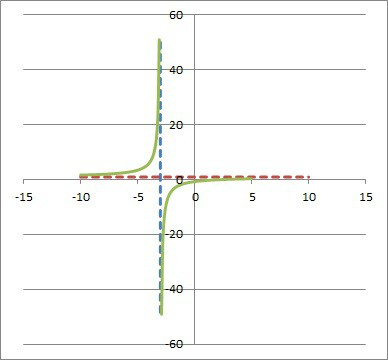Finally, we graph the right half of the rational function R(x) = (x – 2) / (x + 3), for x > -3 (green curve).

If you ever get stuck with your graph, choose a few more values of x, plug them into the rational function R(x), and plot some more points to help you get a better sense of the graph.

## Domain & Asymptotes Of Rational Functions

We can also say a few things about the domain, range, and asymptotes of rational functions.

### What Is The Domain Of A Rational Function?

The domain of a rational function is all real numbers, except the ones that make the denominator zero.  In the example from earlier, the rational function

• R(x) = (x – 2) / (x + 3)

has a domain of R – {-3}, or all real numbers except x = -3, since the denominator of R(x) is zero when x = -3.

[Note: we can also consider complex numbers as the domain of R(x) if we wish to consider them].

### Can A Rational Function Have No Asymptotes?

A rational function can have no asymptotes if the denominator polynomial Q(x) is a constant polynomial – that is, Q(x) = k for some constant value k.

A rational function can have no vertical asymptotes when Q(x) has only imaginary or complex (not real) zeros.

Let’s take a look at some examples of each situation.

#### Example 1: A Rational Function With Constant Denominator & No Asymptotes.

Consider the rational function R(x):

• R(x) = (2x + 1) / 4

So P(x) = 2x + 1 and Q(x) = 4.  In this case, the denominator polynomial Q(x) is constant, and there are no asymptotes, as we can see from the graph below.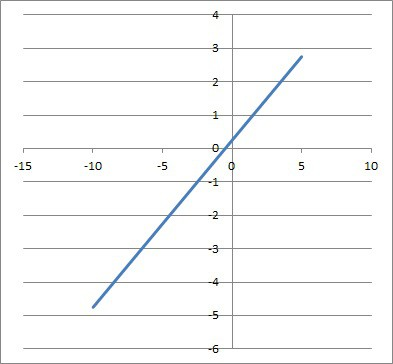The rational function R(x) = (2x + 1) / 4 is a polynomial (specifically, a line). Thus, is has no vertical asymptotes and no horizontal asymptotes.

#### Example 2: A Rational Function With No Vertical Asymptotes.

Consider the rational function R(x):

• R(x) = (3x2) / (x2 + 1)

So P(x) = 3x2 and Q(x) = x2 + 1.  In this case, the denominator polynomial Q(x) is not zero for any real number x (it would be zero for the imaginary numbers x = i and x = -i).

This means there are no vertical asymptotes, but a horizontal asymptote at y = 3 as we can see from the graph below.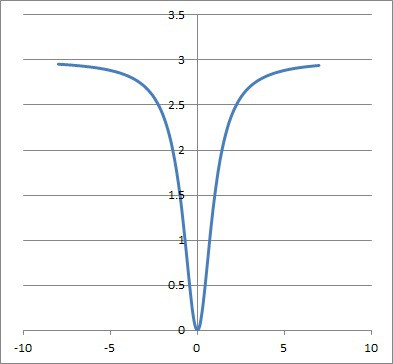The graph of the rational function R(x) = 3x2 / (x2 + 1) has no vertical asymptotes, but it has a horizontal asymptote at y = 3.

### Can A Rational Function Have Two Vertical Asymptotes?

A rational function can have two (or more) vertical asymptotes if the denominator is zero at two or more real values of x.  To find the vertical asymptotes, we might need to factor the denominator Q(x).

#### Example: A Rational Function With Two Vertical Asymptotes

Consider the rational function R(x):

• R(x) = 4x2 / (x2 – 1)

So P(x) = 4x2 and Q(x) = x2 – 1.  In this case, we can factor the denominator polynomial Q(x) as:

• x2 – 1 = (x + 1)(x – 1)

This means that Q(x) = 0 when x = 1 or x = -1.  This means there are two vertical asymptotes at x = 1 and x = -1, along with a horizontal asymptote at y = 4 as we can see from the graph below.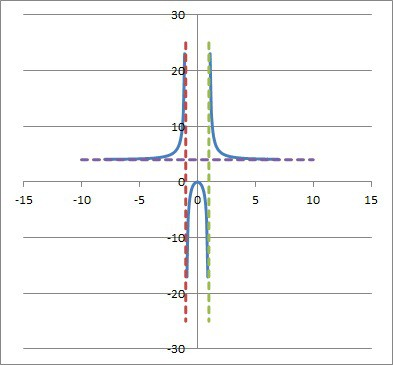The graph of the rational function R(x) = 4x2 / (x2 – 1) (blue curves) has vertical asymptotes at x = -1 (dashed red line) and x = 1 (dashed green line), and also a horizontal asymptote at y = 4 (dashed purple line).

### Can A Rational Function Cross A Vertical Asymptote?

A rational function cannot cross a vertical asymptote.  The reason is that the x-value of a vertical asymptote makes the denominator of the rational function zero.

This means that there is no y-value that corresponds to the x-value of the vertical asymptote (the function is undefined at that x-value).

### Can A Rational Function Have Two Horizontal Asymptotes?

A rational function cannot have two horizontal asymptotes.  A rational function R(x) = P(x) / Q(x) has only one horizontal asymptote:

• If the degree of P(x) is less than the degree of Q(x), then the horizontal asymptote is y = 0.
• If P(x) and Q(x) have the same degree, then the horizontal asymptote is y = a / b, where a is the leading coefficient of P(x) and b is the leading coefficient of Q(x).
• If the degree of P(x) is 1 greater than the degree of Q(x), then there is no horizontal asymptote.  Instead, there is a skew (slant) asymptote, which is the line y = (ax + b) / c, where a and b are the two leading coefficients of P(x) and c is the leading coefficient of Q(x).

### Can A Rational Function Cross A Horizontal Asymptote?

A rational function can cross a horizontal asymptote in some cases.

#### Example: A Rational Function That Crosses A Horizontal Asymptote

Consider the rational function R(x):

• R(x) = (3x2 + 9x + 15) / (x2 – 4)

So P(x) = 3x2 and Q(x) = x2 – 4.  In this case, the horizontal asymptote occurs at y = 3, and there are vertical asymptotes at x = -2 and x = 2.

The graph crosses the horizontal asymptote y = 3 when:

• R(x) = 3
• (3x2 + 9x + 15) / (x2 – 4) = 3
• (x2 + 3x + 5) / (x2 – 4) = 1
• x2 + 3x + 5 = x2 – 4
• 3x + 5 = -4
• 3x = -9
• x = -3

So the graph of R(x) crosses the horizontal asymptote y = 3 at the point (-3, 3), as we can see in the graph below: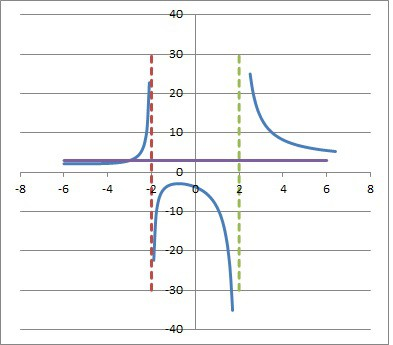The graph of the rational function R(x) = (3x2 +9x + 15) / (x2 – 4) (blue curves) crosses the horizontal asymptote y = 3 (purple line) at x = -3.

## Symmetry & Properties Of Rational Functions

We can also look at the symmetry and other properties of rational functions, such as whether they are even or odd.

### Are Rational Functions Symmetric?

A rational function can have even symmetry, odd symmetry, or neither, depending on the exponents in the numerator and denominator polynomials.

### Can Rational Functions Be Even Or Odd?

A rational function R(x) = P(x) / Q(x) is even if every exponent of every term in P(x) and Q(x) is even.

A rational function R(x) = P(x) / Q(x) is odd if every exponent of every term in P(x) and Q(x) is odd.

Otherwise, R(x) is neither even nor odd.

#### Example 1: Even Rational Function

Consider the rational function R(x):

• R(x) = (x2 + 4) / (x2 – 4)

So P(x) = x2 + 4 and Q(x) = x2 – 4.  In this case, the exponent of every term in P(x) and Q(x) is even (either 2 or 0).

This means that R(x) is even (symmetric about the y-axis), as we can see in the graph below.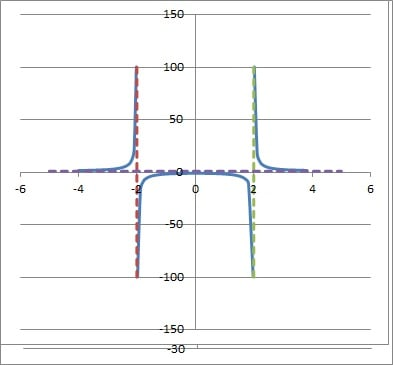The graph of the rational function R(x) = (x2 + 4) / (x2 – 4) (blue curves) has vertical asymptotes at x = -2 (dashed red line) and x = 2 (dashed green line), and also a horizontal asymptote at y = 1 (dashed purple line).

#### Example 2: Odd Rational Function

Consider the rational function R(x):

• R(x) = (x3 – 5x)/ (2x3 + 3x)

So P(x) = x3 – 5x and Q(x) = 2x3 + 3x.  In this case, the exponent of every term in P(x) and Q(x) is even (either 3 or 1).

This means that R(x) is odd (symmetric about the origin), as we can see in the graph below.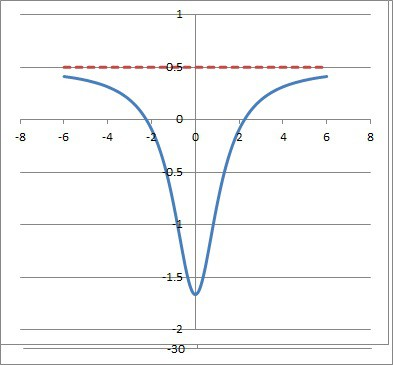The graph of the rational function R(x) = (x3 – 5x) / (2x3 + 3x) (blue curves) has a hole (removable discontinuity) at x = 0 and also a horizontal asymptote at y = 1/2 (dashed red line).

#### Example 3: Rational Function That Is Neither Even Nor Odd

Consider the rational function R(x):

• R(x) = 1 / x

So P(x) = 1 and Q(x) = x.  In this case, the exponent of the single term in P(x) is even (zero) and the exponent of the single term in Q(x) is odd (1).

This means that R(x) is neither even nor odd, as we can see in the graph below.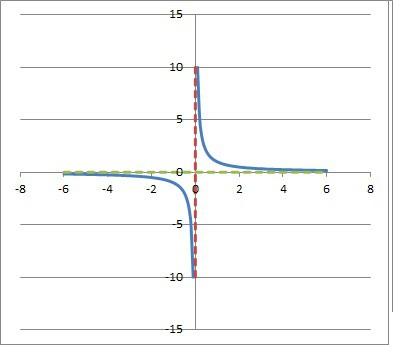Graph of the rational function R(x) = 1 / x (blue curves), which has a vertical asymptote at x = 0 (dashed red line) and a horizontal asymptote at y = 0 (dashed green line).

### Is A Rational Function One To One?

Some rational functions are one to one (injective), but not all.  Remember that in order for a function to be one-to-one, it must pass the horizontal line test.

This means that any horizontal line will intersect the graph of the function at most once.

#### Example 1: A Rational Function That Is One-To-One

Consider the rational function R(x):

• R(x) = 1 / x

So P(x) = 1 and Q(x) = x.  In this case, there is no horizontal line that intersects the graph of R(x) more than once, as we can see in the graph below.The graph of the rational function R(x) = 1 / x (blue curves) is one-to-one, since any horizontal line intersects the graph at most once.

#### Example 2: A Rational Function That Is Not One-To-One

Consider the rational function R(x):

• R(x) = 1 / x2

So P(x) = 1 and Q(x) = x2.  In this case, there are horizontal lines (such as y = 1) that intersect the graph of R(x) more than once (y = 1 intersects at x = 1 and x = -1) as we can see in the graph below.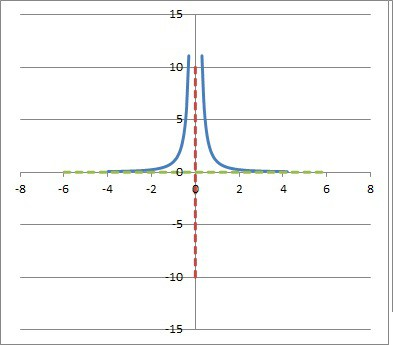The graph of the rational function R(x) = 1 / x2 (blue curves) is not one-to-one, since the horizontal line y = 1 intersects it twice, at x = 1 and x = -1.

### Is A Rational Function Continuous?

A rational function is continuous at every point except where it has a vertical asymptote.  So, a rational function is continuous on its domain: the set of real numbers without the zeros of the denominator polynomial Q(x).

### Are Rational Functions Differentiable?

Rational functions are differentiable at every point except where it has a vertical asymptote.  So, a rational function is differentiable on its domain: the set of real numbers without the zeros of the denominator polynomial Q(x).

To find the derivative of a rational function R(x) = P(x) / Q(x), we use the quotient rule:

• R’(x) = (P’(x)Q(x) – P(x)Q’(x)) / (Q(x))2

## Conclusion

Now you know what a rational function is (and what it is not).  You also know the answers to some common questions about rational functions and some examples to illustrate the ideas.